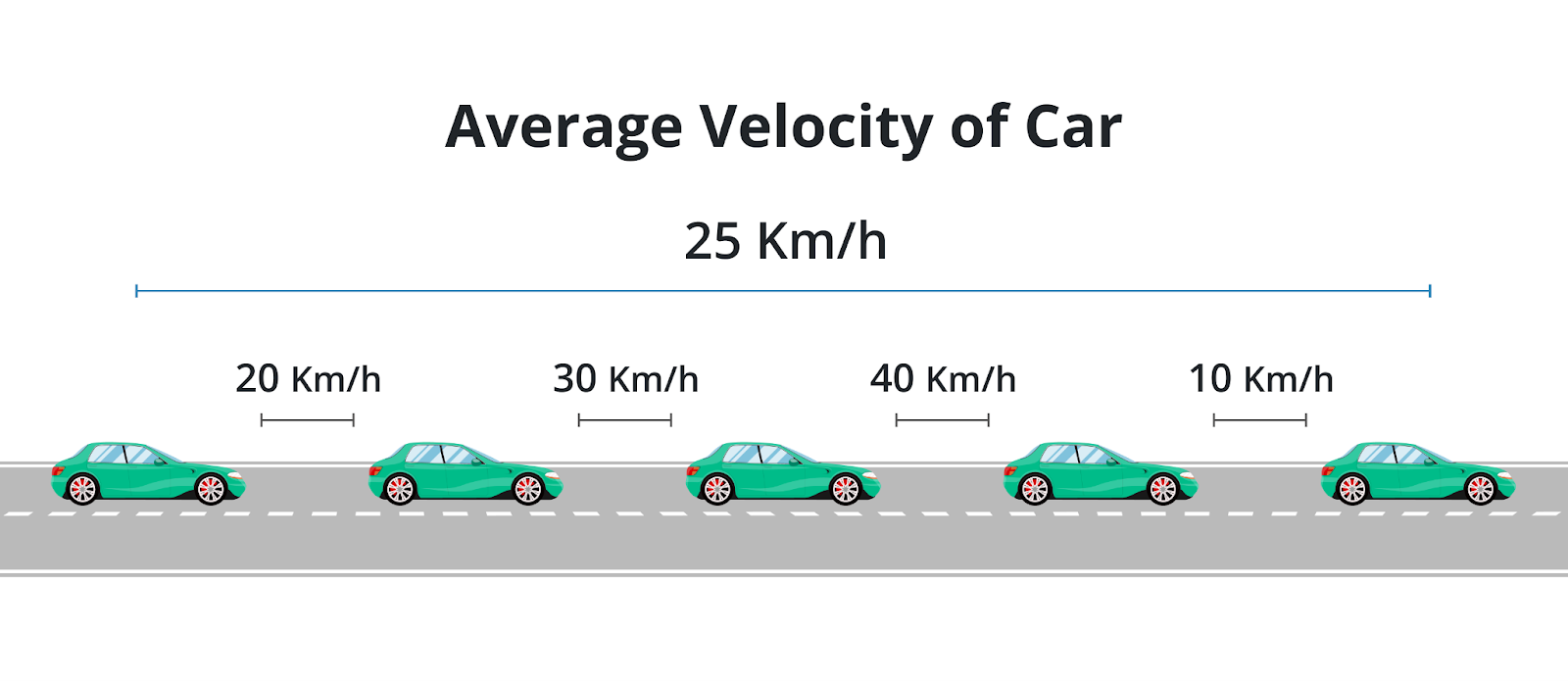# Average Velocity Calculator

Input the values of initial velocity and the final velocity to calculate the average velocity by using the average velocity calculator.

Formula:
Average Velocity (vav) = 1/2 (vi + vf)
ms-1
ms-1

Give Us Feedback

## Average Velocity Calculator

Velocity calculator of physics is an online tool that can calculate average velocity if initial and final velocities are known.

This final velocity calculator can calculate:

• Average velocity
• Initial velocity
• Final velocity

If you want to calculate initial velocity with acceleration and time, check out this initial velocity calculator.

## What is average velocity?

Average velocity can be defined as:

The rate at which an object changes its position from one place to another.

In simple words, it is the distance divided by the time interval for the distance traveled.

The S.I. unit of average velocity is a meter per second (m/s).

## Average velocity formula

The equation to calculate the average velocity is:

va = (vi + vf)/2

Where,

• va is average velocity,
• vi is initial velocity, and
• vf is the final velocity.## How to calculate average velocity?

Want to know how to find average or initial velocity? Let’s find out using an example.

Example:

What is the average velocity of a car if its initial velocity is 5 m/s and final velocity is 15 m/s?

Solution:

Step 1: Identify the values.

vi = 5 m/s

vf = 15 m/s

Step 2: Put the values into the formula.

va = (vi + vf)/2

va = (5 + 15)/2

va = 20/2

va = 10 m/s

### Math Tools